# C Input and Output Basic Quiz

Question 1.

Find the output of the given program?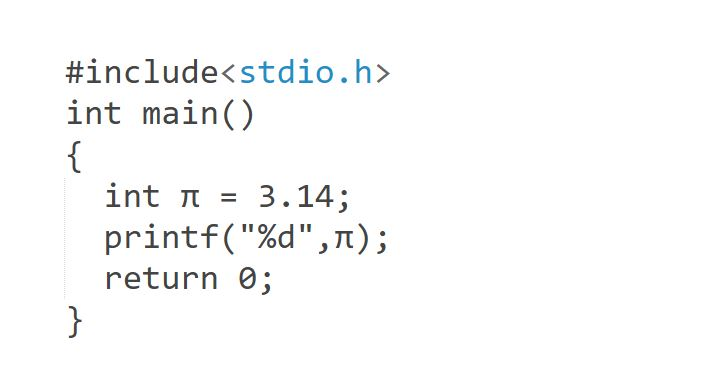• A) Compile-time error
• B) None of these
• C) 3
• D) 3.14
Question 2.

Find the output of the given program?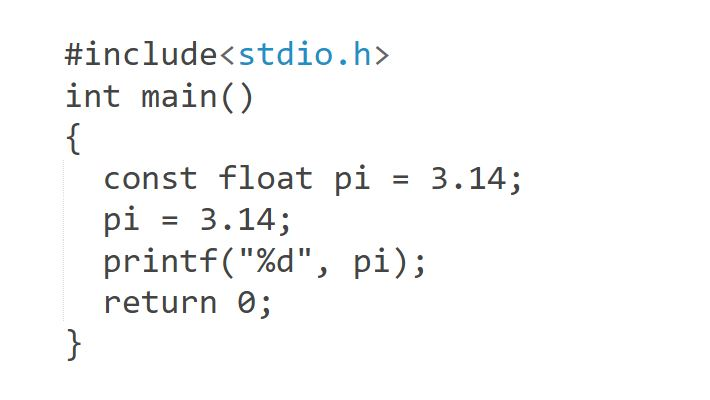• A) Compile-time error
• B) None of these
• C) 3
• D) 3.14
Question 3.

What is the output of the given below program?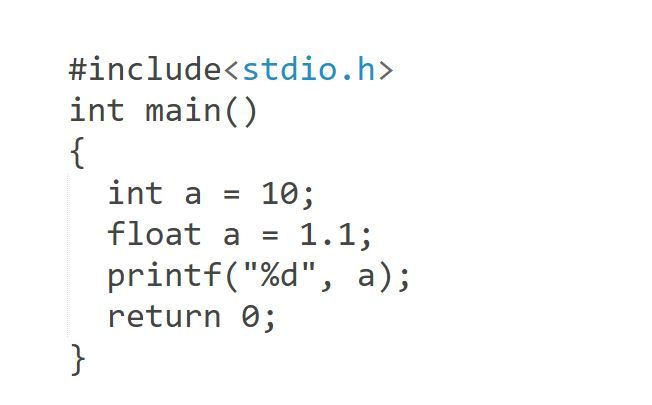• A) 10
• B) 1.1
• C) Compile-time error
• D) 1
Question 4.

Find the output of the given program?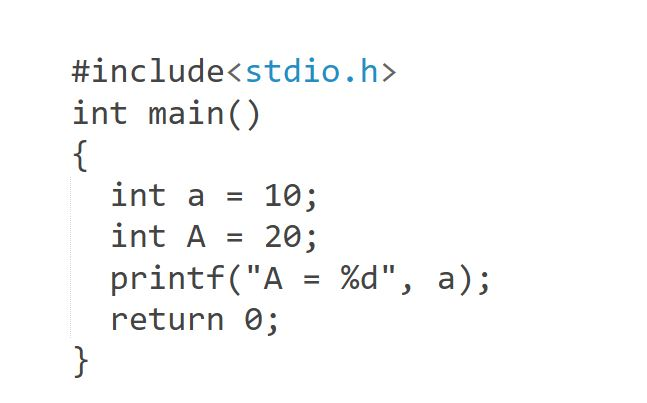• A) 10
• B) a = 10
• C) Compile-time error
• D) A = 10
Question 5.

To print out a and b were given below, which of the following `printf()` statement will you use?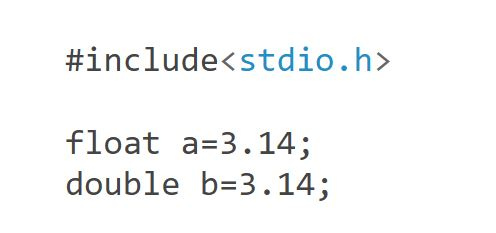• A) printf("%f %lf", a, b);
• B) printf("%Lf %f", a, b);
• C) printf("%Lf %Lf", a, b);
• D) printf("%f %Lf", a, b);
Question 6.

In a call to `printf()`` `function the format specifier %b can be used to print binary equivalent of an integer.

• A) True
• B) False
Question 7.

Can we specify a variable filed width in a scanf() format string?

• A) Yes
• B) No
Question 8.

Point out the error in the program?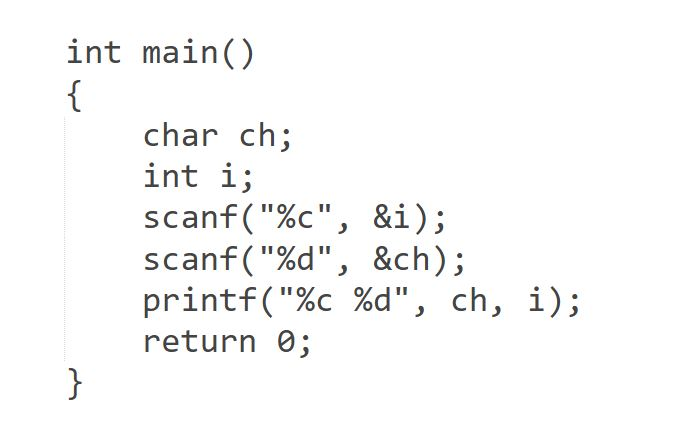• A) Error: suspicious char to in conversion in scanf()
• B) Error: we may not get input for second scanf() statement
• C) No error
• D) None of above
Question 9.

Find the output of the given C program.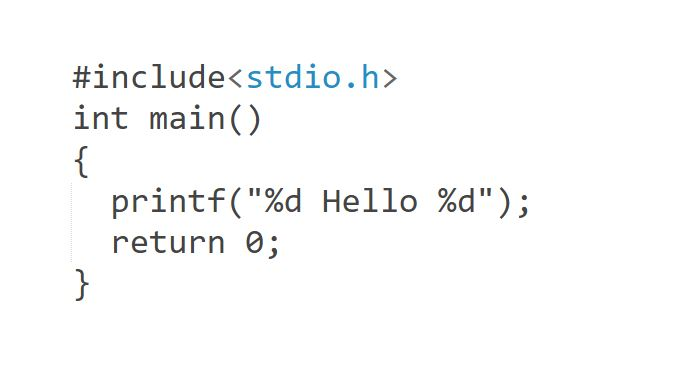• A) None of these
• B) Garbage_value Hello Garbage_value
• C) 1 Hello 1
• D) 0 Hello 0
Question 10.

Find the output of the given C program.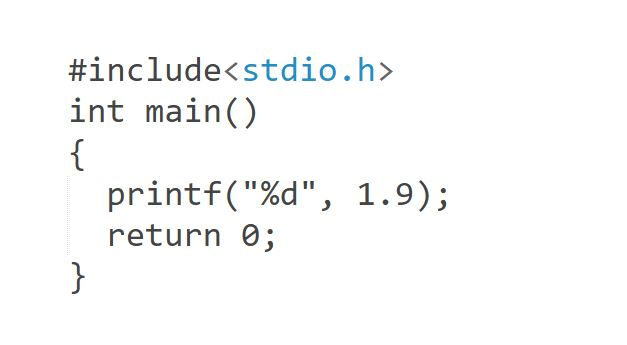• A) garbage value
• B) 1.9
• C) 1
• D) None of these
Recent Articles
Interview Questions
Recent Blogs